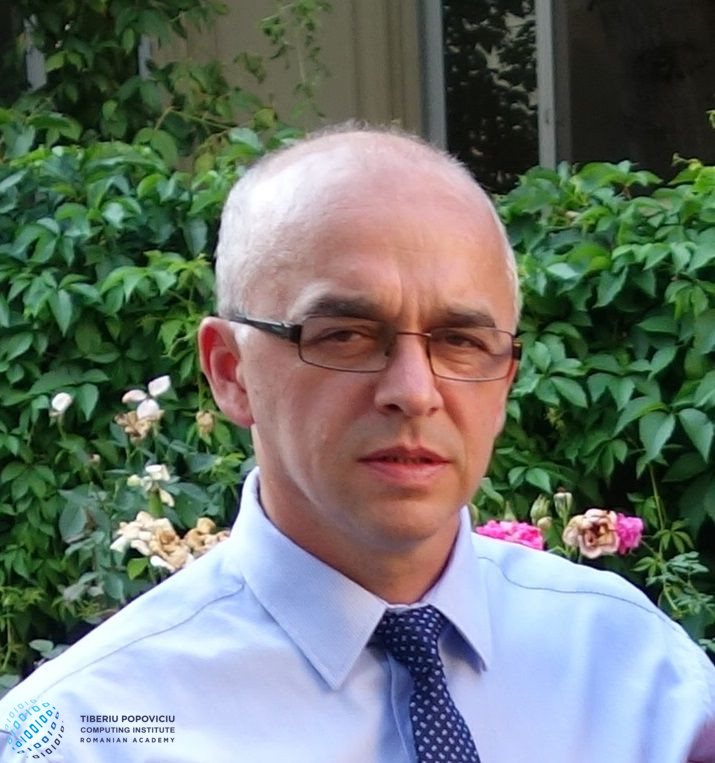Former member of ICTP.

He was formed here as numerical analyst – he has prepared and defended the PhD thesis while working at the Institute, and has published several papers in the field.
After leaving ICTP he has published a (cited) paper in SIAM J. Sci. Comput.

Fields of interest:

• Approximation Theory
• Nonlinear Iterative Methods (Newton, conjugate gradients)
• Numerical Analysis/Numerical Linear Algebra:
– iterative methods for solving linear systems;
– multilevel methods (multigrid, algebraic multigrid);
• Numerical Methods for Solving Differential and Partial Differential Equations:
– finite differences, finite element, wavelets, meshless;
• Parallel Algorithms (MPI)
• Methods for Accelerating the Convergence of Sequences

version: March 12, 2018.

## Simultaneous approximation by Gauss–Weierstrass–Wachnicki operators

Abstract In this note, we spotlight a generalization of Weierstrass integral operators introduced by Eugeniusz Wachnicki. The construction involves modified Bessel functions. The operators are correlated with diffusion equation. Our…